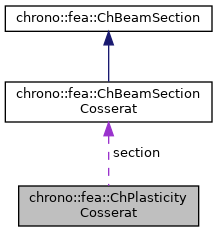chrono::fea::ChPlasticityCosserat Class Referenceabstract

## Description

Base class for plasticity of beam sections of Cosserat type.

This can be shared between multiple beams.

#include <ChBeamSectionCosserat.h>

Inheritance diagram for chrono::fea::ChPlasticityCosserat:[legend]
Collaboration diagram for chrono::fea::ChPlasticityCosserat:[legend]

## Public Member Functions

virtual bool ComputeStressWithReturnMapping (ChVector<> &stress_n, ChVector<> &stress_m, ChVector<> &e_strain_e_new, ChVector<> &e_strain_k_new, ChBeamMaterialInternalData &data_new, const ChVector<> &tot_strain_e, const ChVector<> &tot_strain_k, const ChBeamMaterialInternalData &data)=0

virtual void ComputeStiffnessMatrixElastoplastic (ChMatrixNM< double, 6, 6 > &K, const ChVector<> &strain_e, const ChVector<> &strain_k, const ChBeamMaterialInternalData &data)
Compute the 6x6 tangent material stiffness matrix [Km] = dσ/dε, given actual internal data and deformation and curvature (if needed). More...

virtual void CreatePlasticityData (int numpoints, std::vector< std::unique_ptr< ChBeamMaterialInternalData >> &plastic_data)

## Public Attributes

ChBeamSectionCosseratsection

double nr_yeld_tolerance

int nr_yeld_maxiters

## ◆ ComputeStiffnessMatrixElastoplastic()

 void chrono::fea::ChPlasticityCosserat::ComputeStiffnessMatrixElastoplastic ( ChMatrixNM< double, 6, 6 > & K, const ChVector<> & strain_e, const ChVector<> & strain_k, const ChBeamMaterialInternalData & data )
virtual

Compute the 6x6 tangent material stiffness matrix [Km] = dσ/dε, given actual internal data and deformation and curvature (if needed).

If in plastic regime, uses elastoplastic matrix, otherwise uses elastic. This must be overridden by subclasses if an analytical solution is known (preferred for high performance), otherwise the base behaviour here is to compute [Km] by numerical differentiation calling ComputeStressWithReturnMapping() multiple times.

Parameters
 K 6x6 material stiffness matrix values here strain_e tot strain (deformation part): x= elongation, y and z are shear strain_k tot strain (curvature part), x= torsion, y and z are line curvatures data updated material internal variables, at this point, including {p_strain_e, p_strain_k, p_strain_acc}

## ◆ ComputeStressWithReturnMapping()

 virtual bool chrono::fea::ChPlasticityCosserat::ComputeStressWithReturnMapping ( ChVector<> & stress_n, ChVector<> & stress_m, ChVector<> & e_strain_e_new, ChVector<> & e_strain_k_new, ChBeamMaterialInternalData & data_new, const ChVector<> & tot_strain_e, const ChVector<> & tot_strain_k, const ChBeamMaterialInternalData & data )
pure virtual
Parameters
 stress_n local stress (generalized force), x component = traction along beam stress_m local stress (generalized torque), x component = torsion torque along beam e_strain_e_new updated elastic strain (deformation part) e_strain_k_new updated elastic strain (curvature part) data_new updated material internal variables, at this point, including {p_strain_e, p_strain_k, p_strain_acc} tot_strain_e trial tot strain (deformation part): x= elongation, y and z are shear tot_strain_k trial tot strain (curvature part), x= torsion, y and z are line curvatures data trial material internal variables, at this point, including {p_strain_e, p_strain_k, p_strain_acc}

Implemented in chrono::fea::ChPlasticityCosseratLumped.

The documentation for this class was generated from the following files:
• /builds/uwsbel/chrono/src/chrono/fea/ChBeamSectionCosserat.h
• /builds/uwsbel/chrono/src/chrono/fea/ChBeamSectionCosserat.cpp